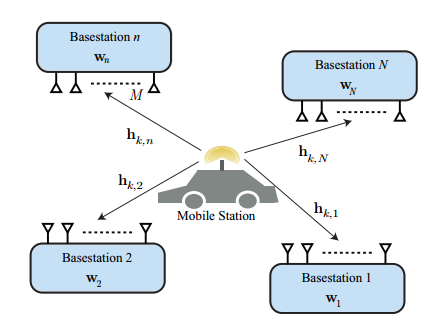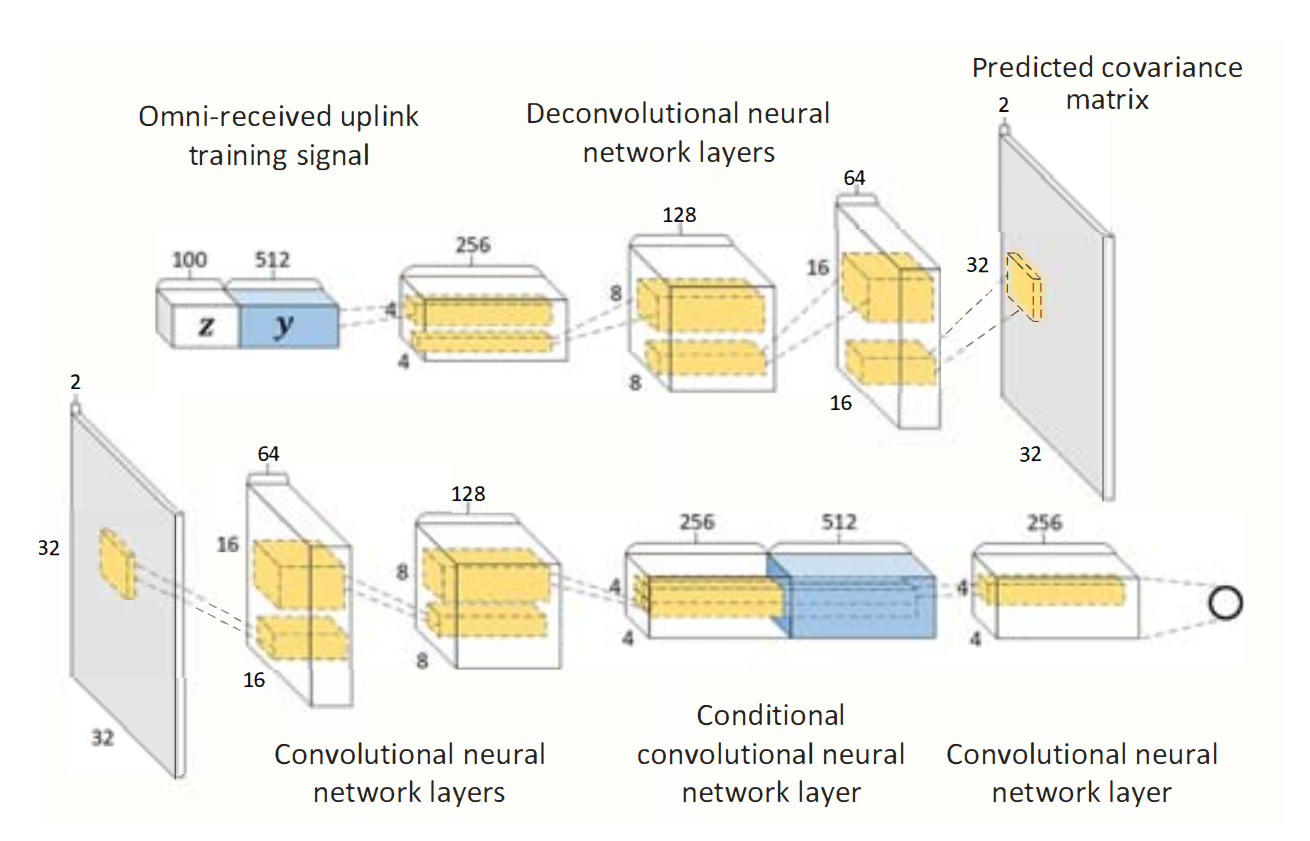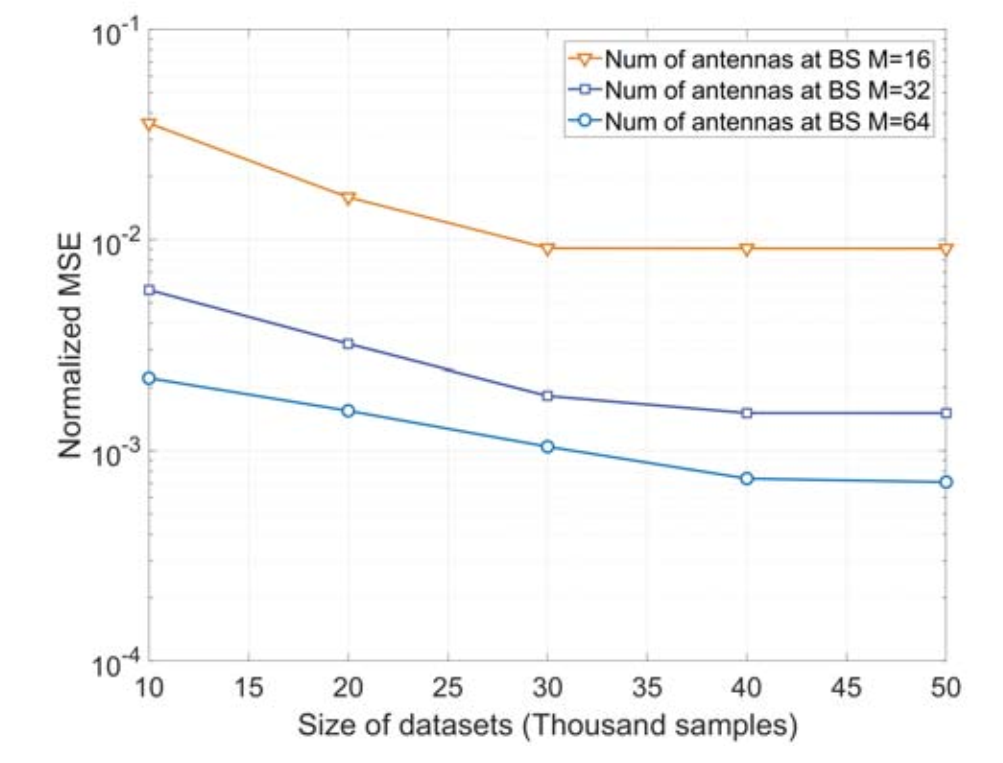# 系统模型## 信道模型

$\boldsymbol{h}_{d, n}=\sqrt{\frac{M}{\rho}} \sum_{\ell=1}^{L} g_{n, \ell} p\left(d T_{s}-\tau_{n, \ell}\right) \mathbf{a}\left(\theta_{n, \ell}\right)$

$\mathbf{h}_{k, n}=\sum_{d=0}^{D-1} \boldsymbol{h}_{d, n} e^{-j \frac{2 \pi k}{K} d}，$

$y_{k, n}=\mathbf{w}_{n}^{T} \mathbf{h}_{k, n} s_{k}+v_{k}$

## 网络相关

• 通过所有基站上收到的pilot， 估计信道。因此，输入向量可写为$\mathbf{y}=\left[y_{1,1}, \cdots, y_{K, 1}, y_{1,2}, \cdots, y_{K, 2}, \cdots, y_{K, N}\right]^{T}$
也就是所有基站所有子载波上的一个pilot的结合。
如同在Deep Learning Coordinated Beamforming for Highly-Mobile Millimeter Wave Systems提出的一样，这篇文章沿用了 **Omni-antenna patterns：

In , the authors showed that when the uplink training pilots are received simultaneously by multiple distributed basestations using omni or quasi-omni antenna patterns, these omni-received signals draw a rich multipath signature for the user location and its interaction with the surrounding environment.

$\mathbf{w}_{n}=[1,0, \dots, 0], \forall n$

• 不需要设计接受波束成形向量了，很方便。

## 稀疏变换

$\mathbf{R}_{\mathbf{h}_{n}}=\mathbb{E}\left[\mathbf{h}_{k, n} \mathbf{h}_{k, n}^{H}\right]$

$\boldsymbol{h}_{d, n}=\mathbf{U}_{\mathrm{BS}} \boldsymbol{g}_{d, n}$
$\mathbf{U}_{\mathrm{BS}}$ 是DFT矩阵。 由于空间中路径数十分有限，因此$\boldsymbol{g}_{d, n}$中绝大部分元素都为0。（这一部分看不懂的同学可以查阅下作者给出的引文《An Overview of Signal Processing Techniques for Millimeter Wave MIMO Systems》）。

$\mathbf{R}_{\mathbf{h}_{n}}=\mathbf{U}_{\mathrm{BS}} \mathbf{R}_{\mathrm{g}_{n}} \mathbf{U}_{\mathrm{BS}}^{H}$

it is much easier to learn the mapping from the omni-received uplink signature y to the virtual channel covariance matrices as compared to the original channel covariance matrix.

# 网络模型• 通过导频序列，生成$\mathbf{R}_{\mathrm{g}_{n}}$。 可以理解为导频通过神经网络，输出了信道预测值。
本文和其他许多基于深度学习的信道估计文章不同， 其他文章往往以准确信道信息为标签，并计算网络输出值与真实值的MSE作为loss，不断训练网络缩小loss， 而本文则是通过GAN的方式来完成训练：
\begin{aligned} \min _{G} \max _{D} L(G, D) =\mathbb{E}_{\mathbf{R}_{\mathbf{\varepsilon}_{n}}}\left[\log D\left(\mathbf{R}_{\mathbf{g}_{n}} | \mathbf{y}\right)\right] +\mathbb{E}_{\mathbf{z}}[\log (1-D(G(\mathbf{z} | \mathbf{y})))] \end{aligned}
这是GAN的经典公式： 先固定生成器G， 训练最优的判别器D来区分真实样本和生成样本。 在这里，分别代表了 真实信道信息和估计（生成）的信道信息。 然后，固定判别器D， 训练生成器，使得估计的信道信息尽可能贴近于真实信道信息。 来回迭代，直至收敛。
GAN相关的推导可以参考这里$G(\mathbf{z} | \mathbf{y}))$表示在接收的导频序列为$\mathbf{y}$的情况下，通过噪声$\mathbf{z}$生成（估计）的$\mathbf{R}_{\mathbf{g}_{n}}$, 也可以表示为$\hat{\mathbf{R}}_{\mathbf{g}_{n}}=G(\mathbf{z}, \mathbf{y})$

# 仿真结果

$\mathrm{NMSE}=\frac{\left\|\hat{\mathbf{R}}_{\mathrm{g}_{\mathrm{n}}}-\mathbf{R}_{\mathrm{g}_{\mathrm{n}}}\right\|_{\mathrm{F}}^{2}}{\left\|\mathbf{R}_{\mathrm{g}_{\mathrm{n}}}\right\|_{\mathrm{F}}^{2}}$#### 零基础深度学习入门：由浅入深理解反向传播算法

07-08

03-112040

#### 基于压缩感知的Massive mmWave Massive MIMO信道估计学习总结04-174万+

#### 搭建你自己的深度通信网络：配置深度学习环境新手向教程

08-152394

#### 利用生成对抗网络保护通信（learning to Protect Communications with Adversarial Neural Cryptography）附代码

04-122246

#### 毫米波蜂窝系统的信道估计及混合预编码

03-253万+

#### 【JSON解析】浅谈JSONObject的使用

11-101万+

#### 信道估计算法

08-248万+

#### 技术人员要拿百万年薪，必须要经历这9个段位

04-22826

#### 深度学习：用生成对抗网络（GAN）来恢复高分辨率（高精度）图片 （附源码，模型与数据集）...

05-072万+

#### 深度通信网络专栏（2）|自编码器：无信道模型的通信系统端到端学习

03-28530

#### 毫米波大规模MIMO的AOA估计

08-30959

#### GAN生成对抗网络综述

02-17778

#### 宽带情况的智能反射面信道估计——arxiv上的最新paper阅读笔记

12-302万+

#### 只因接了一个电话，程序员被骗 30 万！

06-01397

#### 深度通信网络专栏（4）|自编码器：Blind Channel Equalization using Variational Autoencoders

01-0118万+

#### 复习一周，京东+百度一面，不小心都拿了Offer

11-06203

#### 专栏：谈谈我对当下大数据整顿的理解与风控建议

06-302407

#### Python可以这样学（第一季：Python内功修炼）

02-23©️2020 CSDN 皮肤主题: 大白 设计师: CSDN官方博客点击重新获取扫码支付1.余额是钱包充值的虚拟货币，按照1:1的比例进行支付金额的抵扣。
2.余额无法直接购买下载，可以购买VIP、C币套餐、付费专栏及课程。余额充值